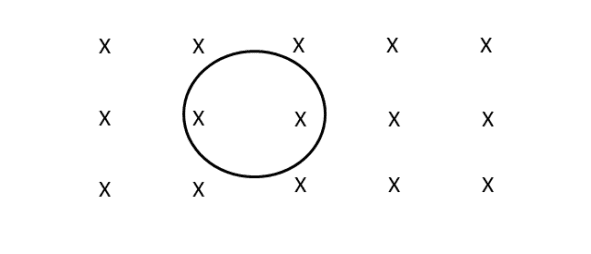# Electromotive force (EMF) of a circular loop wire

Hi, so, I got help from this homework problem by posting it on chegg, but I want clarification.

A circular loop of wire of radius 12.0 cm is placed in a magnetic field directed perpendicular to the plane of the loop and into the page. The field is decreasing at a constant rate of 0.050 T per second. What is the magnitude (in volts) of the emf induced in the loop during this time interval? Experts only please and also please show all work and drawings and be clear!Is this right, and how did he get 1000?

Required magnitude of emf in mV = 1000*pi*(0.12^2)*0.05 = 2.26 mV

Last edited by a moderator:

1000 is multiplied to give answer in mV the answer in volts would be 0.00226 V.

berkeman
Mentor